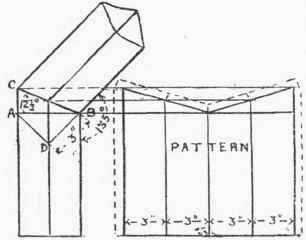If the pipes run diagonally and an elbow is required, as shown in Fig. 28, it will be necessary to first obtain a diagonal of the pipe before the elevation can be drawn. This can be done by setting out the right-angle triangle A, B, D, the sides A D and D B being made equal in length to the diameter of the pipe. The diagonal A B can be calculated, and in the present case where the diameter of the pipe is 3 in. it will come out as follows: -Fig. 28.

AB = 3√2 = 4.24 = 4 1/4in.

The height of A C can be found by either of the methods explained for the last elbow.

If the seam is down the back edge the girth of the pattern will be made up by four widths, each equal to the diameter of the pipe.

For elbows of this description it should be observed that the cut at top of pattern is simply two straight lines. The pattern for a square elbow can be marked out in the same manner; but in this the height A C will be the same length as a diagonal of the pipe.Fig. 29.